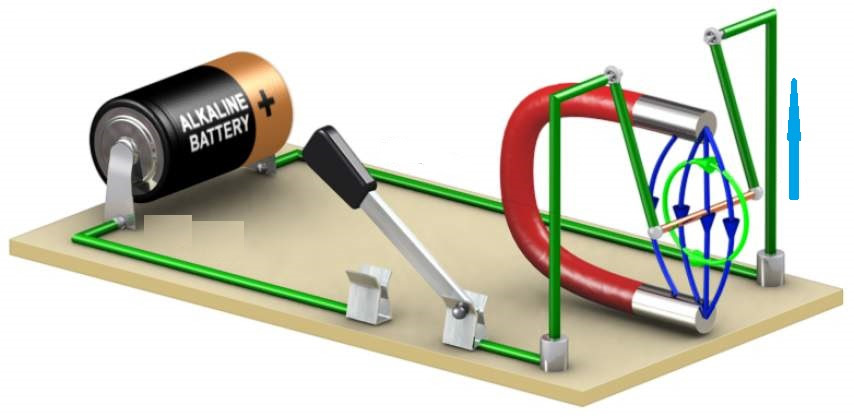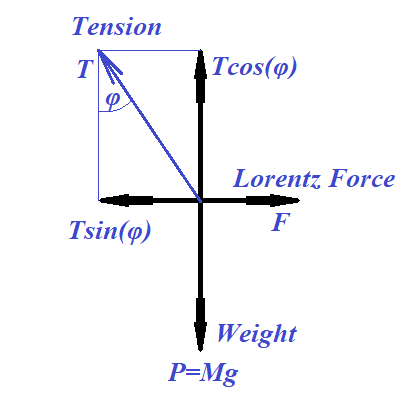## Monday, May 25, 2020

### Unizor - Physics4Teens - Electromagnetism - Magnetic Field - Problems 1

Notes to a video lecture on http://www.unizor.com

Magnetism - Lorentz Force - Problems 1

Problem 1a

Consider the experiment pictured below.A copper wire (yellow) of resistance R is connected to a battery with voltage U and is swinging on two connecting wires (green) in a magnetic field of a permanent magnet.

All green connections are assumed light and their weight can be ignored.
Also ignored should be their electric resistance. Assume the uniformity
of the magnetic field of a magnet with magnetic field lines directed
vertically and perpendicularly to a copper wire.

The mass of a copper wire is M and its length is L.

The experiment is conducted in the gravitational field with a free fall acceleration g.

The magnetic field exerts the Lorentz force onto a wire pushing it
horizontally out from the field space, so green vertical connectors to a
copper wire make angle φ with vertical.

What is the intensity of a magnetic field B?

SolutionT·cos(φ) = M·g

T = M·g/cos(φ)

F = T·sin(φ) = M·g·tan(φ)

I = U/R

F = I·L·B = U·L·B/R

M·g·tan(φ) = U·L·B/R

B = M·R·g·tan(φ)/(U·L)

Problem 1b

An electric point-charge q travels with a speed v along a wire of length L.

What is the value of the equivalent direct electric current I in the wire that moves the same amount of electricity per unit of time?

What is the Lorentz force exerted onto a charge q, if it moves in a uniform magnetic field of intensity B perpendicularly to the field lines with a speed v.

Solution

Let T be the time of traveling from the beginning to the end of a wire.

T = L/v

I = q/T = q·v/L

F = I·L·B = q·v·B

Notice, the Lorentz force onto a wire in case of only a point-charge
running through it does not depend on the length of a wire, as it is
applied only locally to a point-charge, not an entire wire. Would be the
same if a particle travels in vacuum with a magnetic field present.

Problem 1c

An electric point-charge q of mass m enters a uniform magnetic field of intensity B perpendicularly to the field lines with a speed v.Suggest some reasoning (rigorous proof is difficult) that the trajectory
of this charge should be a circle and determine the radius of this
circle.

Solution

The Lorentz force exerted on a point-charge q, moving with speed v perpendicularly to force lines of a permanent magnetic field of intensity B, is directed always perpendicularly to a trajectory of a charge and equals to F=q·v·B (see previous problem).

Since the Lorentz force is always perpendicular to trajectory, the linear speed v of a point-charge remains constant, while its direction always curves toward the direction of the force. Constant linear speed v
means that the magnitude of the Lorentz force is also constant and only
direction changes to be perpendicular to a trajectory of a charge.

According to the Newton's Second Law, this force causes acceleration a=F/m,
which is a vector of constant magnitude, since the Lorentz force has
constant magnitude and always perpendicular to a trajectory, since the
force causing this acceleration is always perpendicular to a trajectory.

So, the charge moves along a trajectory with constant linear speed and
constant acceleration always directed perpendicularly to a trajectory.

Every smooth curve at any point on an infinitesimal segment around this
point can be approximated by a small circular arc of some radius (radius of curvature) with a center at some point (center of curvature). If a curve of a trajectory on an infinitesimal segment is approximated by a circle of some radius R, the relationship between a radius, linear speed and acceleration towards a center of this circle (centripetal acceleration), according to kinematics of rotational motion, is

a = v²/R

Therefore, R = v²/a

Since v and a are constant, the radius of a curvature R
is constant, which is a good reason towards locally circular character
of the motion of a charge. It remains to be proven that the center of
the locally circular motion does not change its location, but this is a
more difficult task, which we will omit.

Hence,

R = v²/a = m·v²/F =

= m·v²/q·v·B = m·v/q·B